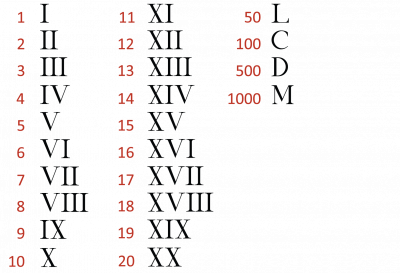The Romans used capital letters to write their numbers. Roman numbers are called numerals. The Romans put different letters together to make different numbers.If you put a letter after a larger one it means you add it

Here is an example:

LV = 55

This means 50 (L) + 5 (V) = 55

50 + 5 = 55

If you put a letter before a larger one it means you take it away

Here is an example:

XC = 90

This means you take 10 (X) away from 100 (C)

100 - 10 = 90

Now that you have read some examples of Roman numerals, let's start the activities!